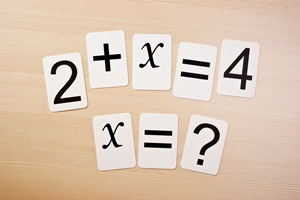Mental math strategies young students should know
FRIDAY, JUNE 26, 2015 10:19 AMAs early as first grade, the Common Core asks students to be able to do mental math. Older students have to make calculations in their heads all the time, so learning mental math early helps students develop a fluency in the simple operations they need to know. If students are unable to do basic addition, subtraction, multiplication and division in their heads by the time they start learning more complex mathematical concepts, they may find themselves struggling to keep up. Here are a few strategies younger children may be able to use to do mental math:

Making 10
The first mental math strategy is very commonly used by adults and students alike for addition and subtraction. The method involves finding the nearest 10 when making a calculation, since 10 is one of the easiest numbers to work with in math. Here's an example: 8+9=X.To do this problem mentally, students can first make 10, by adding 2 to 8. Then, after subtracting that 2 from 9, students are left with a very easy math problem: 10+7=17.

Although this may seem like a lot of work for smaller numbers, it's a useful tool when doing larger calculations as well. For example, what's the sum of 285 and 30? Students can make 10 by adding 5, then "make 100" by adding another 10. So, after adding 15 total, they're left with: 300+15=315.

The same strategy can be used to subtract - it's all about manipulating the numbers you're given to find an equation that's easier to make sense of.

Near doubles
Near doubles is another addition strategy for younger students. The idea is that doubling a number is an easy calculation to make in your head, so it's a good mental method if you're working with two numbers that are near each other. For instance, 11+13 may not be as easy a calculation as 11+11+2 for students who already know that 11 doubled is 22. So, 22+2=24.

Double and half
Multiplying and dividing mentally can be a little bit trickier for students. One strategy many educators teach their pupils is to double one number and halve the other when you're working with difficult numbers. Here's an example: What is the product of 4 times 14? Instead of trying to solve the equation with those numbers, students can double 4 and halve 14 to make them easier to work with. So, the new equation would be 8x7=56.RELATED ARTICLES All About TerraNova 3 Testing Teaching gifted kids in normal classes Helping your kids continue learning this summer More..
 NEWS CATEGORIES NEWS ARCHIVE